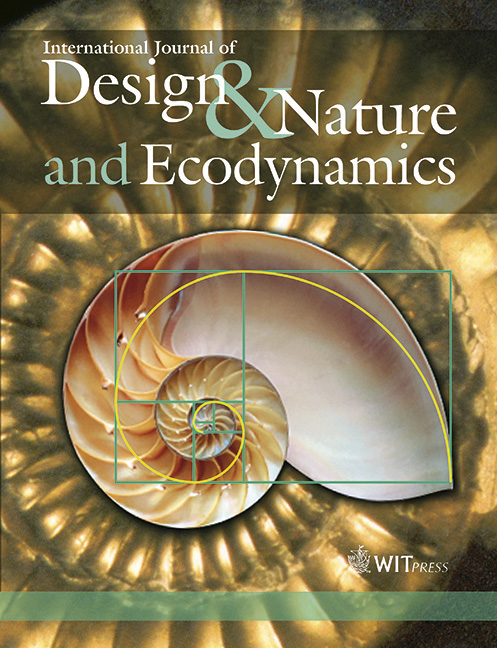WIT Press

MODELING OF PULSATING PERIPHERAL BIOHEAT TRANSFER USING FRACTIONAL CALCULUS AND CONSTRUCTAL THEORY

Price

Free (open access)Volume

Volume 1 (2006), Issue 1

Pages

15

Page Range

18 - 33

Paper DOI

10.2495/D&N-V1-N1-18-33

WIT Press

Author(s)

R. MAGIN, S. BOREGOWDA & C. DEODHAR

Abstract

Fractional calculus provides novel mathematical tools for modeling physical and biological processes. The bioheat equation is often used as a first order model of heat transfer in biological systems. In this paper we describe the formulation of the bioheat transfer in one dimension in terms of the fractional order differentiation with respect to time. The solution to the resulting fractional order partial differential equation reflects the interaction of the system with the dynamics of its response to the pulsed surface or volume heating and cooling. The resulting expression for the heat flux in terms of the fractional order is used to derive an expression for the depth of thermal penetration. This depth of thermal penetration, expressed as a function of time, is optimized using constructal theory, which in turn leads to the determination of the optimal time of pulsating (on–off) heating and cooling. The advantage of using the fractional heat flux expression is demonstrated by comparing the results w

Keywords

bioheat transfer, constructal theory, diffusion, fractals, fractional calculus, laser surgery, modeling